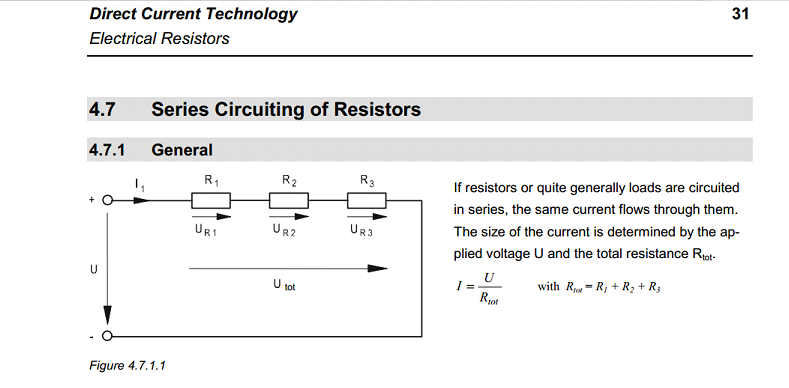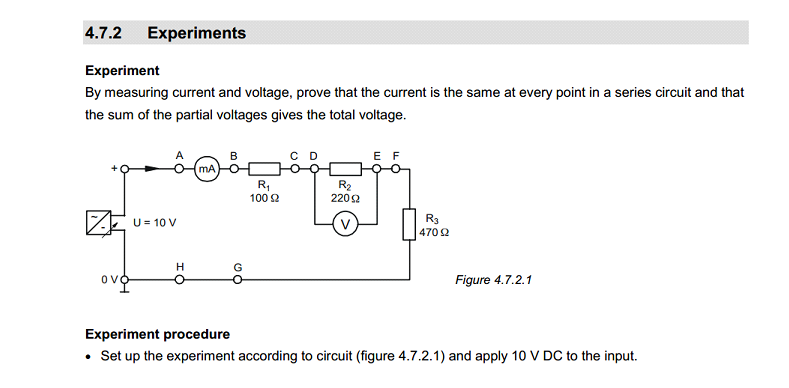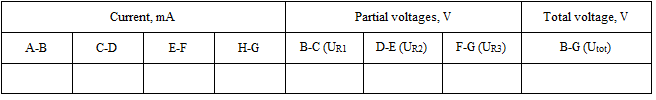Maths
Engineering
Series Circuiting of resistors
Author
Gintautas Stonys
Target Group
gymnasium
Brief Description
Exercices of serial resistors

Share
• Series Circuiting of Resistors

GeneralIf resistors or quite generally loads are circuited in series, the same current flows through them. The size of the current is determined by the ap plied voltage U and the total resistance Rtot:

Rtot = R1+R2+R3

A partial voltage is applied to each individual resistor. The sum of all the partial voltages gives the total resistance.

Utot= UR1+UR2+UR3

Experiment

By measuring current and voltage, prove that the current is the same at every point in a series circuit and that the sum of the partial voltages gives the total voltage.Experiment procedure

-Set up the experiment according to circuit (figure 2) and apply 10 V DC to the input.

-Break the connections between A-B, C-D, E-F and G-H one after another and measure the current through the series circuit between the test points.

-Then measure the partial voltages at test points B-C, D-E, F-G as well as the total voltage at test point B-G.

-Break the connections between point A and power supply and measure the resistance between point A and H.

Table 1Question 1: How do the partial voltages behave in relation to the corresponding resistance value?

Question 2: Are the measured resistivity directly different from the calculated according to the formulas?

Question 3: How to determine the resistance between points A and B as indirect measurements?

## Related files

 Connection of electrical measuring instruments-ilovepdf-compressed 152 KB Series Circuiting of Resistors 378 KB Solution_Series Circuiting of Resistors_T 497 KB
If you have tried out a lesson, please fill the next form to help us to improve it: